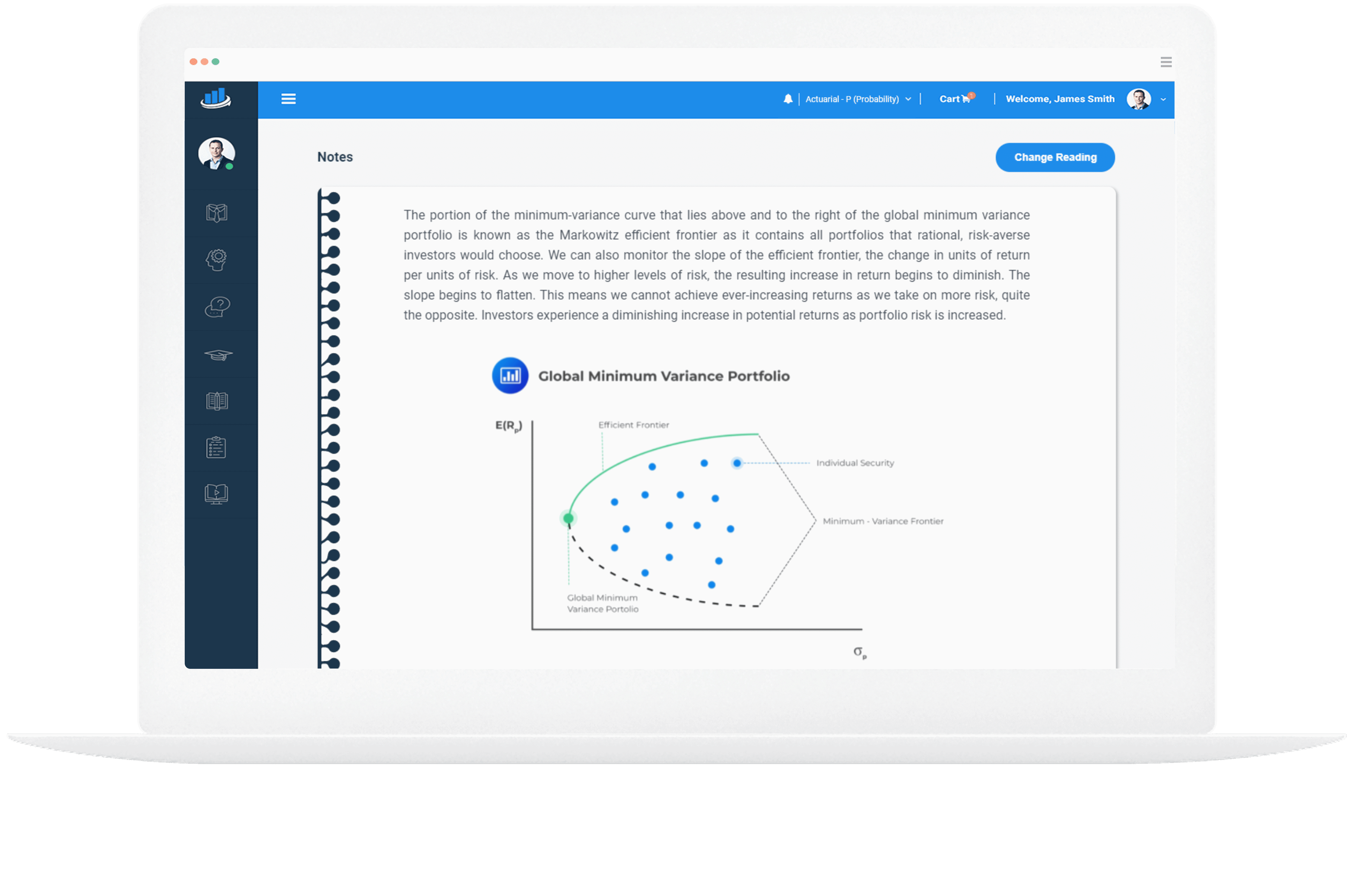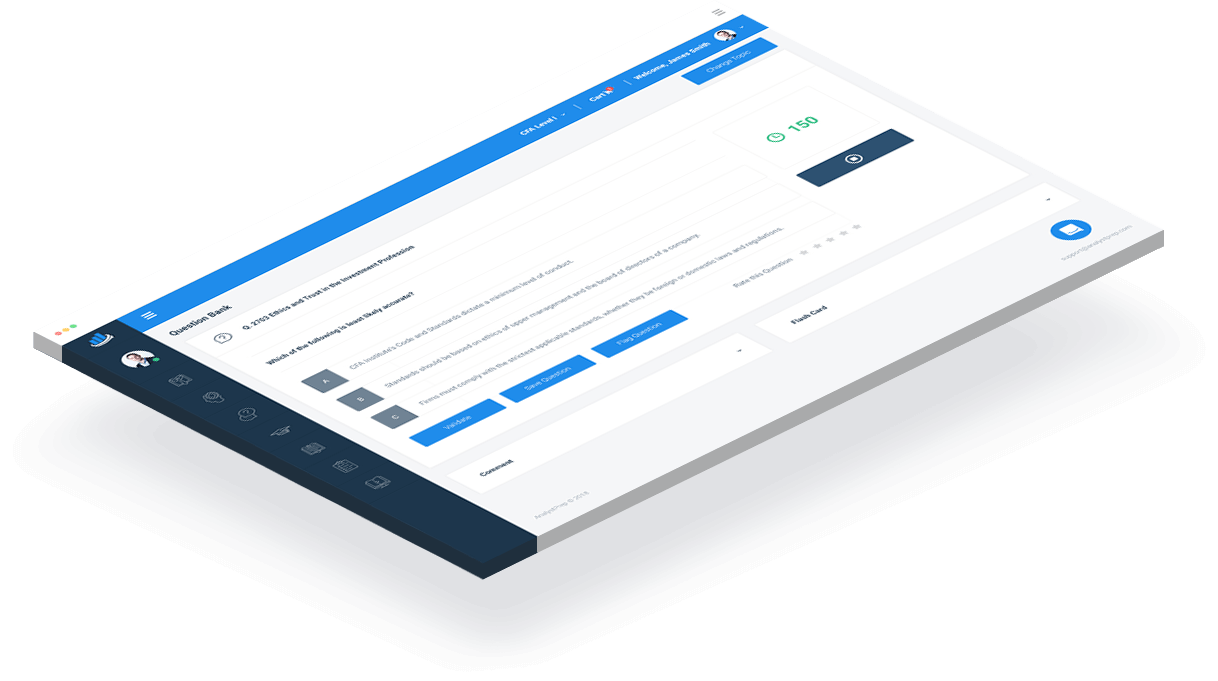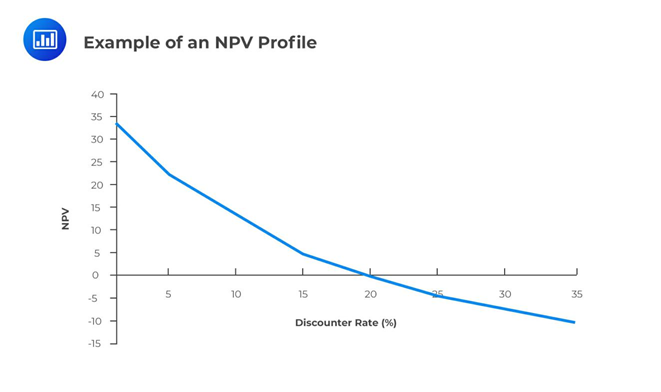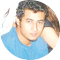Study Materials for 2023 CFA®, FRM®, Actuarial, GMAT® and EA® Exams

# Study Notes for Level I of the CFA® Exam

### Every Learning Outcome Statement (LOS) Summarized with Question Examples

The Level I study course consists of a vast amount of material contained in a 5,000-page CFA Institute official books that make up the curriculum. There are 10 topic areas that vary in emphasis and weight at each exam level. The amount of information to memorize and understand can be overwhelming. For this reason, we’ve produced comprehensive study notes in an easy-to-read format to help you learn efficiently and effectively.

According to CFA Institute, candidates require a minimum of 300 hours of study at each level and at least 6 months to prepare for Level I of the CFA exam. With statistics showing the pass rate hovering at 40% over the past 10 years, you need to perform better than the average candidate to secure a pass. In a 2015 CFA Institute survey, more than 60% of candidates admitted to using third-party preparation materials in addition to reading the assigned books.

4.5  Million
50  Thousand
# 1  Rated
###### CFA PREPARATION PLATFORM BY REVIEW WEBSITES## Why Candidates Love AnalystPrep's Study Notes

Our study notes are designed and written by CFA charterholders to help you understand core concepts covered in the CFA exams in the most efficient way. We’ve produced summaries of each chapter from the latest CFA Program curriculum with clear, detailed explanations to help you understand difficult concepts and speed up your learning.

Spread out across the topics are handy tips and tricks to help you understand concepts faster. Our end-of-LOS questions help you test your understanding of key concepts and build confidence. What’s more, our instructors are always ready to chip in with additional explanations whenever you need them.

A comprehensive formula sheet and Financial Reporting and Analysis ratio sheet are included as quick reference guides and visual resources for the various formulas and ratios you need to memorize.

## This isn't a Sprint

Many candidates have likened the CFA® exam experience to a marathon. We understand how exhausting it can get and want to help you get to the finishing line.

#### Stay on Course with our Study Plans

Plan a study schedule to fit your needs and busy lifestyle and stick to it. We’ll help you stay organized with our proposed one-month or two-month study plan and identify the chapters you should concentrate on. Remember, the chapters are not equally weighted in the evaluation.

Sign up for our coaching package for 5 hours of 1-on-1 coaching and a personalized study plan tailored to your needs.

#### Get Support Whenever you Need It

Our premium members enjoy the privilege of AnalystPrep’s community support. The experts from our support team are here to answer any of your exam related questions within 24 hours so you can stay on track and on schedule with your study plan.

Plus, our in-app forum connects you to a community of other CFA Program candidates, CFA charterholders, and AnaylstPrep founders, wherever you are and whenever you wish. This way, you stay motivated and engaged as you make the journey to your Level I CFA exam.### Example Learning Objective from AnalystPrep's CFA Level 1 Study Notes

#### LOS 28b: demonstrate the use of net present value (NPV) and internal rate of return (IRR) in allocating capital and describe the advantages and disadvantages of each method.

Several important decision criteria are used to evaluate capital investments. The two most comprehensive and well-understood measures of whether or not a project is profitable are the net present value (NPV) and the internal rate of return (IRR). Other measures include the payback period, discounted payback period, average accounting rate of return (AAR), and the profitability index (PI).

## Net Present Value (NPV)

The net present value (NPV)  of a project is the potential change in wealth resulting from the project after accounting for the time value of money.  The NPV for a project with one investment outlay made at the start of the project is defined as the present value of the future after-tax cash flows minus the investment outlay.

$$\mathrm{NPV}=\sum_{t=1}^{n} \frac{C F_{t}}{(1+r)^{t}} \text { – Outlay }$$

Where:

$$CF_t$$ = After-tax cash flow at time t

$$r$$ = Required rate of return for the investment

$$\text{Outlay}$$ = Investment cash flow at time zero

Many projects have cash flow patterns in which outflows occur not only at the start of the project (at time = 0) but also at future dates. In these instances, a better formula to use is:

• to invest in the project if NPV > 0;
• not to invest in the project if NPV < 0; and
• stay indifferent if NPV = 0.

In other words, positive NPV investments are wealth increasing, while negative NPV investments are wealth decreasing.

Suppose Company A is considering an investment of $100 million in a capital expansion project that will return after-tax cash flows of$20 million per year for the first 3 years and another 33 million in year 4, the final year of the project. If the required rate of return for the project is 8%, what would the NPV be, and should the company undertake this project? \begin{align*} \mathrm{NPV} &=\frac{20}{1.08^{1}}+\frac{20}{1.08^{2}}+\frac{20}{1.08^{3}}+\frac{33}{1.08^{4}}-100 \\ &=18.519+17.147+15.877+24.256-100 \\ &=-\ 24.201 \text { million } \end{align*} Since the NPV < 0, the project should not be undertaken. ## Internal Rate of Return The internal rate of return (IRR) is the discount rate that makes the net present value (NPV) of all cash flows from a particular project equal to zero. For a project with one initial outlay, the IRR is the discount rate that makes the present value of the future after-tax cash flows equal to the investment outlay. The IRR solves the equation: $$\sum_{t=1}^{n} \frac{C F_{t}}{(1+I R R)^{t}}-\text { Outlay }=0$$ It looks very much like the NPV equation except that the discount rate is the IRR instead of $$r$$, the required rate of return. Discounted at the IRR, the NPV is equal to zero. The decision rule for the IRR is: • to invest in the project if the IRR exceeds the required rate of return for the project, i.e., invest if IRR > $$r$$; and • not to invest if IRR < $$r$$. In instances where the outlays for a project occur at times other than time 0, a more general form of the IRR equation is: $$\sum_{t=0}^{n} \frac{C F_{t}}{(1+I R R)^{t}}=0$$ #### Example: IRR of a project Here is a follow-up on the above NPV example. If company A is considering an investment of100 million in a capital expansion project that will return after-tax cash flows of $20 million per year for the first 3 years and another$33 million in year 4, the final year of the project, what is the IRR for this project and should it be undertaken given that the required rate of return for the project is 8%?

Solve IRR in the following equation:

$$-100+\frac{20}{(1+I R R)^{1}}+\frac{20}{(1+I R R)^{2}}+\frac{20}{(1+I R R)^{3}}+\frac{33}{(1+I R R)^{4}}=0$$

The solution can be arrived at through trial and error. However, a simpler approach is to use a financial calculator:

Press the Cash Flow [CF] key to open the cash flow register. The calculator should read CF0=, which tells you to enter the cash flow for time 0. Since you need to send cash out of the company to make the initial $100 investment, this value has to be negative. Type in -100 for CF0, and hit the [ENTER] key. ### Step 2: Entering the Cash Inflows Next, enter the cash flow values for the subsequent periods. This is done by hitting the down arrow once. The calculator should read CF1=. Type in the amount for the first cash flow, 20, and hit [ENTER]. The calculator should now say C01=20. To enter cash flow from Year 2, hit the down arrow twice. The calculator should read CF2=. If it says F1=, hit the down arrow one more time. Type in the second year’s cash flow, 20, and hit [Enter]. The calculator should read CF2=20. Hit the down arrow twice again and do the same thing for the third cash flow period, CF3. Do this once more and for the last time to enter the last cash flow, 33. ### Step 3: Calculating the IRR Once the cash flow values have been fed into the calculator, you are ready to calculate the IRR. To do this, press the [IRR] key. The screen will read IRR=0.000. To display the IRR value for the data set, press the [CPT] key at the top left corner of the calculator. If you have followed this process correctly, the calculator will display the correct IRR. The IRR is computed to be -2.626%. Since -2.626% < 8%, the project should not be undertaken. Simply computing a project’s NPV and IRR to determine which of several projects to undertake is not always as straightforward as it seems. The IRR and NPV can produce different ranking outcomes whenever mutually exclusive projects are involved. Other challenges may occur. ## Graphical Illustration The NPV Profile is a graphical illustration of a project’s NPV graphed as a function of various discount rates. The NPV values are graphed on the vertical or y-axis, while the discount rates are graphed on the horizontal or x-axis.• The graph crosses the y-axis (vertical axis) when the discount rate = 0%; and • The graph crosses the x-axis (horizontal axis) when the NPV = 0 and the discount rate is the IRR. ## NPV and IRR Comparison For independent, conventional projects, the decision rules for the NPV and IRR will both draw the same conclusion on whether to invest or not. However, in the case of two mutually exclusive projects, sometimes the decision rules will draw different conclusions. For example, project X might have a larger NPV than project Y, but project Y might have a larger IRR. This conflict usually stems from differences in the cash flows of the two projects, which leads to a different ranking between the NPV and IRR. Whenever this conflict arises, the NPV and not the IRR should be used to select which project to invest in. Another circumstance that may cause mutually exclusive projects to be ranked differently according to NPV and IRR criteria is the scale or size of the project. ## Multiple IRR and No IRR Problem It is quite possible, although rare, for a project to have more than one IRR or no IRR at all. Multiple IRRs, however, cannot occur for conventional projects which have outlay followed by cash inflows, but they may occur for non-conventional projects which have cash flows which change signs (negative, positive) more than once during the project’s life. The net present value (NPV) and the internal rate of return (IRR) are both techniques that can be used by financial institutions or individuals when making major investment decisions. Each method has its strengths and weaknesses. However, the net present value method comes out on top, and here’s why. When dealing with independent projects, both NPV and IRR will yield the same investment decisions. By independent, we mean that deciding to invest in one project does not rule out or affect investment in the other project. However, the challenge comes when the projects are mutually exclusive. If two or more projects are mutually exclusive, the decision to invest in one project precludes investment in all the others. With such projects, the IRR method may provide misleading results if used in isolation. ## Shortcomings of IRR As seen, there are some problems associated with the IRR method: • The method assumes that all proceeds from a project are immediately reinvested in projects offering a rate of return equal to the IRR – this is very difficult in practice. • It gives different rankings when the projects under comparison have different scales. • Sometimes, the method may not provide a unique solution, especially when a project has a mixture of positive and negative cash flows during its productive life. ### Question 1 You have been provided the following cash flows for a capital project: $$\begin{array}{c|c|c|c|c|c|c} \text { Year } & 0 & 1 & 2 & 3 & 4 & 5 \\ \hline \text { Cash flow }(\) & -50,000 & 10,000 & 10,000 & 15,000 & 15,000 & 15,000 \end{array}$$ Given a required rate of return of 8 percent, the NPV and IRR of the project are closest to: 1. NPV:$1,023; IRR: 10.64%.
2. NPV: $974; IRR: 8.68%. 3. NPV:$2,400; IRR: 7.12%.

Solution

\begin{align*} \text{NPV}&=-50,000+\frac{10,000}{1.08^{1}}+\frac{10,000}{1.08^{2}}+\frac{15,000}{1.08^{3}}+\frac{15,000}{1.08^{4}}+\frac{15,000}{1.08^{5}} \\ &=-50,000+9259.26+8573.39+11,907.48++11,025.45+10,208.75 \\ &=\ 974.33 \text { million } \end{align*}

### Question 2

In an NPV profile, the point at which the profile crosses the x-axis is best described as the:

1. project’s IRR.
2. point at which the NPV is highest.
3. point at which the discount rate = 0% and the NPV is the sum of the undiscounted cash flows for the project.

Solution

At the horizontal axis, the NPV = 0, and by definition, this occurs whenever the discount rate is the IRR.

### Question 3

Suppose you have three independent projects – X, Y, and Z. Assume that the hurdle rate is 12% for all three projects. Their NPVs and IRRs are shown below.

$$\begin{array}{c|c|c|c} & \textbf { Project X } & \textbf { Project Y } & \textbf { Project Z } \\ \hline \text { NPV } & \ 20,000 & \ 21,400 & \ 23,000 \\ \text { IRR } & 20 \% & 32 \% & 18 \% \end{array}$$

Assuming the projects are mutually exclusive, which of the following is the most economically feasible project?

1. Z
2. X
3. Y

Solution

$$\begin{array}{c|c|c|c} & \textbf { Project } \mathbf{X} & \textbf { Project Y } & \textbf { Project } \mathbf{Z} \\ \hline \text { NPV } & \ 20,000 & \ 21,400 & \ 23,000 \\ \text { IRR } & 20 \% & 32 \% & 18 \% \\ \text { Decision } & \text { Accept } & \text { Accept } & \text { Accept } \end{array}$$

If the IRR criteria is used, all the three projects would be accepted because they would all increase shareholders’ wealth. Their NPVs are all positive, and again, the three are all acceptable.

However, if the projects are mutually exclusive, then only one project would be chosen. If one were to pick one project based on internal rates of return of the projects, then one would go for Y. This is because its IRR is the highest compared to the other projects.

This decision would be wrong when we consider the sizes of the NPVs of the projects. While Y has the highest IRR, its NPV is lower than that of Z. The best decision would be to go for the project with the highest NPV, and that is project Z. Therefore, if projects are mutually exclusive, the NPV method should be applied.

### CFA® Program

#### Level I Study Packages by AnalystPrep

AnalystPrep's study packages for the Level I of the CFA Exam start as low as $199. Add video lessons and study notes for only$150 extra.

###### / 12-month access
• Question Bank
• CBT Mock Exams
• Performance Tracking Tools
##### Unlimited Package (All 3 Levels)
###### 699
• Question Banks for all three Levels of the CFA Exam
• Mock Exams for all three Levels of the CFA Exam
• Performance Tracking Tools
• Video Lessons for all three Levels of the CFA Exam
• Study Notes for all three Levels of the CFA Exam
• Essential Review Summaries

### Testimonials

“Thanks to your program I passed the first level of the CFA exam, as I got my results today. You guys are the best. I actually finished the exam with 45 minutes left in [the morning session] and 15 minutes left in [the afternoon session]… I couldn’t even finish with more than 10 minutes left in the AnalystPrep mock exams so your exams had the requisite difficulty level for the actual CFA exam.”###### James B.

“I loved the up-to-date study materials and Question bank. If you wish to increase your chances of CFA exam success on your first attempt, I strongly recommend AnalystPrep.”###### Jose Gary

“Before I came across this website, I thought I could not manage to take the CFA exam alongside my busy schedule at work. But with the up-to-date study material, there is little to worry about. The Premium package is cheaper and the questions are well answered and explained. The question bank has a wide range of examinable questions extracted from across the whole syllabus. Thank you so much for helping me pass my first CFA exam.”###### Brian Masibo

“Good Day!
I cleared FRM Part I (May-2018) with 1.1.1.1. Thanks a lot to AnalystPrep and your support.
Regards,”@AnalystPrep provided me with the necessary volume of questions to insure I went into test day having in-depth knowledge of every topic I would see on the exams.”###### Justin T.

“Great study materials and exam-standard questions. In addition, their customer service is excellent. I couldn’t have found a better CFA exam study partner.”###### Joshua Brown

“I bought their FRM Part 1 package and passed the exam. Their customer support answered all of my questions when I had problems with what was written in the curriculum. I’m planning to use them also for the FRM Part 2 exam and Level I of the CFA exam.”###### Zubair Jatoi

“I bought the FRM exam premium subscription about 2 weeks ago. Very good learning tool. I contacted support a few times for technical questions and Michael was very helpful.”[]